GeeksforGeeks App
Open AppBrowser
Continue

# PySpark Join Types – Join Two DataFrames

In this article, we are going to see how to join two dataframes in Pyspark using Python. Join is used to combine two or more dataframes based on columns in the dataframe.

Syntax: dataframe1.join(dataframe2,dataframe1.column_name ==  dataframe2.column_name,”type”)

where,

1. dataframe1 is the first dataframe
2. dataframe2 is the second dataframe
3. column_name is the column which are matching in both the dataframes
4. type is the join type we have to join

## Python3

 `# importing module``import` `pyspark`` ` `# importing sparksession from pyspark.sql module``from` `pyspark.sql ``import` `SparkSession`` ` `# creating sparksession and giving an app name``spark ``=` `SparkSession.builder.appName(``'sparkdf'``).getOrCreate()`` ` `# list  of employee data``data ``=` `[[``"1"``, ``"sravan"``, ``"company 1"``],``        ``[``"2"``, ``"ojaswi"``, ``"company 1"``], ``        ``[``"3"``, ``"rohith"``, ``"company 2"``],``        ``[``"4"``, ``"sridevi"``, ``"company 1"``], ``        ``[``"5"``, ``"bobby"``, ``"company 1"``]]`` ` `# specify column names``columns ``=` `[``'ID'``, ``'NAME'``, ``'Company'``]`` ` `# creating a dataframe from the lists of data``dataframe ``=` `spark.createDataFrame(data, columns)`` ` `dataframe.show()`

Output: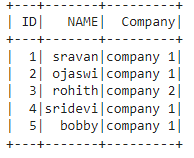## Python3

 `# importing module``import` `pyspark`` ` `# importing sparksession from pyspark.sql module``from` `pyspark.sql ``import` `SparkSession`` ` `# creating sparksession and giving an app name``spark ``=` `SparkSession.builder.appName(``'sparkdf'``).getOrCreate()`` ` `# list  of employee data``data1 ``=` `[[``"1"``, ``"45000"``, ``"IT"``],``         ``[``"2"``, ``"145000"``, ``"Manager"``],``         ``[``"6"``, ``"45000"``, ``"HR"``],``         ``[``"5"``, ``"34000"``, ``"Sales"``]]`` ` `# specify column names``columns ``=` `[``'ID'``, ``'salary'``, ``'department'``]`` ` `# creating a dataframe from the lists of data``dataframe1 ``=` `spark.createDataFrame(data1, columns)`` ` `dataframe1.show()`

Output: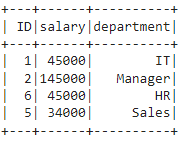## Inner join

This will join the two PySpark dataframes on key columns, which are common in both dataframes.

Syntax: dataframe1.join(dataframe2,dataframe1.column_name ==  dataframe2.column_name,”inner”)

Example:

## Python3

 `# importing module``import` `pyspark`` ` `# importing sparksession from pyspark.sql module``from` `pyspark.sql ``import` `SparkSession`` ` `# creating sparksession and giving an app name``spark ``=` `SparkSession.builder.appName(``'sparkdf'``).getOrCreate()`` ` `# list  of employee data``data ``=` `[[``"1"``, ``"sravan"``, ``"company 1"``], ``        ``[``"2"``, ``"ojaswi"``, ``"company 1"``],``        ``[``"3"``, ``"rohith"``, ``"company 2"``],``        ``[``"4"``, ``"sridevi"``, ``"company 1"``], ``        ``[``"5"``, ``"bobby"``, ``"company 1"``]]`` ` `# specify column names``columns ``=` `[``'ID'``, ``'NAME'``, ``'Company'``]`` ` `# creating a dataframe from the lists of data``dataframe ``=` `spark.createDataFrame(data, columns)`` ` `# list  of employee data``data1 ``=` `[[``"1"``, ``"45000"``, ``"IT"``], ``         ``[``"2"``, ``"145000"``, ``"Manager"``], ``         ``[``"6"``, ``"45000"``, ``"HR"``],``         ``[``"5"``, ``"34000"``, ``"Sales"``]]`` ` `# specify column names``columns ``=` `[``'ID'``, ``'salary'``, ``'department'``]`` ` `# creating a dataframe from the lists of data``dataframe1 ``=` `spark.createDataFrame(data1, columns)`` ` ` ` `# inner join on two dataframes``dataframe.join(dataframe1,``               ``dataframe.``ID` `=``=` `dataframe1.``ID``,``               ``"inner"``).show()`

Output: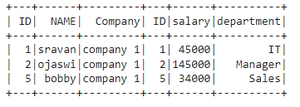## Full Outer Join

This join joins the two dataframes with all matching and non-matching rows, we can perform this join in three ways

Syntax:

• outer: dataframe1.join(dataframe2,dataframe1.column_name ==  dataframe2.column_name,”outer”)
• full: dataframe1.join(dataframe2,dataframe1.column_name ==  dataframe2.column_name,”full”)
• fullouter: dataframe1.join(dataframe2,dataframe1.column_name ==  dataframe2.column_name,”fullouter”)

### Example 1: Using outer keyword

In this example, we are going to perform outer join based on the ID column in both dataframes.

## Python3

 `# importing module``import` `pyspark`` ` `# importing sparksession from pyspark.sql module``from` `pyspark.sql ``import` `SparkSession`` ` `# creating sparksession and giving an app name``spark ``=` `SparkSession.builder.appName(``'sparkdf'``).getOrCreate()`` ` `# list  of employee data``data ``=` `[[``"1"``, ``"sravan"``, ``"company 1"``],``        ``[``"2"``, ``"ojaswi"``, ``"company 1"``],``        ``[``"3"``, ``"rohith"``, ``"company 2"``],``        ``[``"4"``, ``"sridevi"``, ``"company 1"``],``        ``[``"5"``, ``"bobby"``, ``"company 1"``]]`` ` `# specify column names``columns ``=` `[``'ID'``, ``'NAME'``, ``'Company'``]`` ` `# creating a dataframe from the lists of data``dataframe ``=` `spark.createDataFrame(data, columns)`` ` `# list  of employee data``data1 ``=` `[[``"1"``, ``"45000"``, ``"IT"``],``         ``[``"2"``, ``"145000"``, ``"Manager"``], ``         ``[``"6"``, ``"45000"``, ``"HR"``],``         ``[``"5"``, ``"34000"``, ``"Sales"``]]`` ` `# specify column names``columns ``=` `[``'ID'``, ``'salary'``, ``'department'``]`` ` `# creating a dataframe from the lists of data``dataframe1 ``=` `spark.createDataFrame(data1, columns)`` ` ` ` `# full outer join on two dataframes``dataframe.join(dataframe1, ``               ``dataframe.``ID` `=``=` `dataframe1.``ID``, ``               ``"outer"``).show()`

Output: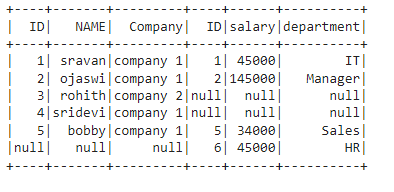### Example 2: Using full keyword

In this example, we are going to perform outer join using full keyword based on ID column in both dataframes.

## Python3

 `# importing module``import` `pyspark`` ` `# importing sparksession from pyspark.sql module``from` `pyspark.sql ``import` `SparkSession`` ` `# creating sparksession and giving an app name``spark ``=` `SparkSession.builder.appName(``'sparkdf'``).getOrCreate()`` ` `# list  of employee data``data ``=` `[[``"1"``, ``"sravan"``, ``"company 1"``], ``        ``[``"2"``, ``"ojaswi"``, ``"company 1"``], ``        ``[``"3"``, ``"rohith"``, ``"company 2"``],``        ``[``"4"``, ``"sridevi"``, ``"company 1"``],``        ``[``"5"``, ``"bobby"``, ``"company 1"``]]`` ` `# specify column names``columns ``=` `[``'ID'``, ``'NAME'``, ``'Company'``]`` ` `# creating a dataframe from the lists of data``dataframe ``=` `spark.createDataFrame(data, columns)`` ` `# list  of employee data``data1 ``=` `[[``"1"``, ``"45000"``, ``"IT"``],``         ``[``"2"``, ``"145000"``, ``"Manager"``],``         ``[``"6"``, ``"45000"``, ``"HR"``],``         ``[``"5"``, ``"34000"``, ``"Sales"``]]`` ` `# specify column names``columns ``=` `[``'ID'``, ``'salary'``, ``'department'``]`` ` `# creating a dataframe from the lists of data``dataframe1 ``=` `spark.createDataFrame(data1, columns)`` ` ` ` `# full outer join on two dataframes``dataframe.join(dataframe1,``               ``dataframe.``ID` `=``=` `dataframe1.``ID``, ``               ``"full"``).show()`

Output: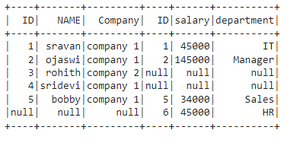### Example 3: Using fullouter keyword

In this example, we are going to perform outer join using full outer based on ID column in both dataframes.

## Python3

 `# importing module``import` `pyspark`` ` `# importing sparksession from pyspark.sql module``from` `pyspark.sql ``import` `SparkSession`` ` `# creating sparksession and giving an app name``spark ``=` `SparkSession.builder.appName(``'sparkdf'``).getOrCreate()`` ` `# list  of employee data``data ``=` `[[``"1"``, ``"sravan"``, ``"company 1"``], ``        ``[``"2"``, ``"ojaswi"``, ``"company 1"``], ``        ``[``"3"``, ``"rohith"``, ``"company 2"``],``        ``[``"4"``, ``"sridevi"``, ``"company 1"``], ``        ``[``"5"``, ``"bobby"``, ``"company 1"``]]`` ` `# specify column names``columns ``=` `[``'ID'``, ``'NAME'``, ``'Company'``]`` ` `# creating a dataframe from the lists of data``dataframe ``=` `spark.createDataFrame(data, columns)`` ` `# list  of employee data``data1 ``=` `[[``"1"``, ``"45000"``, ``"IT"``], ``         ``[``"2"``, ``"145000"``, ``"Manager"``],``         ``[``"6"``, ``"45000"``, ``"HR"``],``         ``[``"5"``, ``"34000"``, ``"Sales"``]]`` ` `# specify column names``columns ``=` `[``'ID'``, ``'salary'``, ``'department'``]`` ` `# creating a dataframe from the lists of data``dataframe1 ``=` `spark.createDataFrame(data1, columns)`` ` ` ` `# full outer join on two dataframes``dataframe.join(dataframe1, ``               ``dataframe.``ID` `=``=` `dataframe1.``ID``,``               ``"fullouter"``).show()`

Output:## Left Join

Here this join joins the dataframe by returning all rows from the first dataframe and only matched rows from the second dataframe with respect to the first dataframe. We can perform this type of join using left and leftouter.

Syntax:

• left: dataframe1.join(dataframe2,dataframe1.column_name ==  dataframe2.column_name,”left”)
• leftouter: dataframe1.join(dataframe2,dataframe1.column_name ==  dataframe2.column_name,”leftouter”)

### Example 1: Perform left join

In this example, we are going to perform left join using the left keyword based on the ID column in both dataframes.

## Python3

 `# importing module``import` `pyspark`` ` `# importing sparksession from pyspark.sql module``from` `pyspark.sql ``import` `SparkSession`` ` `# creating sparksession and giving an app name``spark ``=` `SparkSession.builder.appName(``'sparkdf'``).getOrCreate()`` ` `# list  of employee data``data ``=` `[[``"1"``, ``"sravan"``, ``"company 1"``], ``        ``[``"2"``, ``"ojaswi"``, ``"company 1"``], ``        ``[``"3"``, ``"rohith"``, ``"company 2"``],``        ``[``"4"``, ``"sridevi"``, ``"company 1"``], ``        ``[``"5"``, ``"bobby"``, ``"company 1"``]]`` ` `# specify column names``columns ``=` `[``'ID'``, ``'NAME'``, ``'Company'``]`` ` `# creating a dataframe from the lists of data``dataframe ``=` `spark.createDataFrame(data, columns)`` ` `# list  of employee data``data1 ``=` `[[``"1"``, ``"45000"``, ``"IT"``], ``         ``[``"2"``, ``"145000"``, ``"Manager"``], ``         ``[``"6"``, ``"45000"``, ``"HR"``],``         ``[``"5"``, ``"34000"``, ``"Sales"``]]`` ` `# specify column names``columns ``=` `[``'ID'``, ``'salary'``, ``'department'``]`` ` `# creating a dataframe from the lists of data``dataframe1 ``=` `spark.createDataFrame(data1, columns)`` ` ` ` `# left join on two dataframes``dataframe.join(dataframe1,``               ``dataframe.``ID` `=``=` `dataframe1.``ID``, ``               ``"left"``).show()`

Output: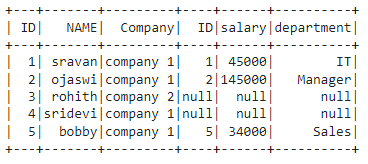### Example 2: Perform leftouter join

In this example, we are going to perform left join  using leftouter keyword based on the ID column in both dataframes

## Python3

 `# importing module``import` `pyspark`` ` `# importing sparksession from pyspark.sql module``from` `pyspark.sql ``import` `SparkSession`` ` `# creating sparksession and giving an app name``spark ``=` `SparkSession.builder.appName(``'sparkdf'``).getOrCreate()`` ` `# list  of employee data``data ``=` `[[``"1"``, ``"sravan"``, ``"company 1"``],``        ``[``"2"``, ``"ojaswi"``, ``"company 1"``],``        ``[``"3"``, ``"rohith"``, ``"company 2"``],``        ``[``"4"``, ``"sridevi"``, ``"company 1"``],``        ``[``"5"``, ``"bobby"``, ``"company 1"``]]`` ` `# specify column names``columns ``=` `[``'ID'``, ``'NAME'``, ``'Company'``]`` ` `# creating a dataframe from the lists of data``dataframe ``=` `spark.createDataFrame(data, columns)`` ` `# list  of employee data``data1 ``=` `[[``"1"``, ``"45000"``, ``"IT"``], ``         ``[``"2"``, ``"145000"``, ``"Manager"``], ``         ``[``"6"``, ``"45000"``, ``"HR"``],``         ``[``"5"``, ``"34000"``, ``"Sales"``]]`` ` `# specify column names``columns ``=` `[``'ID'``, ``'salary'``, ``'department'``]`` ` `# creating a dataframe from the lists of data``dataframe1 ``=` `spark.createDataFrame(data1, columns)`` ` `# left join on two dataframes``dataframe.join(dataframe1, ``               ``dataframe.``ID` `=``=` `dataframe1.``ID``, ``               ``"leftouter"``).show()`

Output## Right Join

Here this join joins the dataframe by returning all rows from the second dataframe and only matched rows from the first dataframe with respect to the second dataframe. We can perform this type of join using right and rightouter.

Syntax:

• right: dataframe1.join(dataframe2,dataframe1.column_name ==  dataframe2.column_name,”right”)
• rightouter: dataframe1.join(dataframe2,dataframe1.column_name ==  dataframe2.column_name,”rightouter”)

### Example 1: Perform right join

In this example, we are going to perform right join using the right keyword based on ID column in both dataframes.

## Python3

 `# importing module``import` `pyspark`` ` `# importing sparksession from pyspark.sql module``from` `pyspark.sql ``import` `SparkSession`` ` `# creating sparksession and giving an app name``spark ``=` `SparkSession.builder.appName(``'sparkdf'``).getOrCreate()`` ` `# list  of employee data``data ``=` `[[``"1"``, ``"sravan"``, ``"company 1"``], ``        ``[``"2"``, ``"ojaswi"``, ``"company 1"``],``        ``[``"3"``, ``"rohith"``, ``"company 2"``],``        ``[``"4"``, ``"sridevi"``, ``"company 1"``],``        ``[``"5"``, ``"bobby"``, ``"company 1"``]]`` ` `# specify column names``columns ``=` `[``'ID'``, ``'NAME'``, ``'Company'``]`` ` `# creating a dataframe from the lists of data``dataframe ``=` `spark.createDataFrame(data, columns)`` ` `# list  of employee data``data1 ``=` `[[``"1"``, ``"45000"``, ``"IT"``], ``         ``[``"2"``, ``"145000"``, ``"Manager"``], ``         ``[``"6"``, ``"45000"``, ``"HR"``],``         ``[``"5"``, ``"34000"``, ``"Sales"``]]`` ` `# specify column names``columns ``=` `[``'ID'``, ``'salary'``, ``'department'``]`` ` `# creating a dataframe from the lists of data``dataframe1 ``=` `spark.createDataFrame(data1, columns)`` ` `# right join on two dataframes``dataframe.join(dataframe1, ``               ``dataframe.``ID` `=``=` `dataframe1.``ID``, ``               ``"right"``).show()`

Output: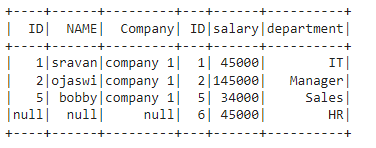### Example 2: Perform rightouter join

In this example, we are going to perform the right join using rightouter keyword based on the ID column in both dataframes.

## Python3

 `# importing module``import` `pyspark`` ` `# importing sparksession from pyspark.sql module``from` `pyspark.sql ``import` `SparkSession`` ` `# creating sparksession and giving an app name``spark ``=` `SparkSession.builder.appName(``'sparkdf'``).getOrCreate()`` ` `# list  of employee data``data ``=` `[[``"1"``, ``"sravan"``, ``"company 1"``], ``        ``[``"2"``, ``"ojaswi"``, ``"company 1"``], ``        ``[``"3"``, ``"rohith"``, ``"company 2"``],``        ``[``"4"``, ``"sridevi"``, ``"company 1"``],``        ``[``"5"``, ``"bobby"``, ``"company 1"``]]`` ` `# specify column names``columns ``=` `[``'ID'``, ``'NAME'``, ``'Company'``]`` ` `# creating a dataframe from the lists of data``dataframe ``=` `spark.createDataFrame(data, columns)`` ` `# list  of employee data``data1 ``=` `[[``"1"``, ``"45000"``, ``"IT"``], ``         ``[``"2"``, ``"145000"``, ``"Manager"``], ``         ``[``"6"``, ``"45000"``, ``"HR"``],``         ``[``"5"``, ``"34000"``, ``"Sales"``]]`` ` `# specify column names``columns ``=` `[``'ID'``, ``'salary'``, ``'department'``]`` ` `# creating a dataframe from the lists of data``dataframe1 ``=` `spark.createDataFrame(data1, columns)`` ` `# right join on two dataframes``dataframe.join(dataframe1,``               ``dataframe.``ID` `=``=` `dataframe1.``ID``, ``               ``"rightouter"``).show()`

Output:## Leftsemi join

This join will all rows from the first dataframe and return only matched rows from the second dataframe

Syntax: dataframe1.join(dataframe2,dataframe1.column_name ==  dataframe2.column_name,”leftsemi”)

Example: In this example, we are going to perform leftsemi join using leftsemi keyword based on the ID column in both dataframes.

## Python3

 `# importing module``import` `pyspark`` ` `# importing sparksession from pyspark.sql module``from` `pyspark.sql ``import` `SparkSession`` ` `# creating sparksession and giving an app name``spark ``=` `SparkSession.builder.appName(``'sparkdf'``).getOrCreate()`` ` `# list  of employee data``data ``=` `[[``"1"``, ``"sravan"``, ``"company 1"``],``        ``[``"2"``, ``"ojaswi"``, ``"company 1"``],``        ``[``"3"``, ``"rohith"``, ``"company 2"``],``        ``[``"4"``, ``"sridevi"``, ``"company 1"``], ``        ``[``"5"``, ``"bobby"``, ``"company 1"``]]`` ` `# specify column names``columns ``=` `[``'ID'``, ``'NAME'``, ``'Company'``]`` ` `# creating a dataframe from the lists of data``dataframe ``=` `spark.createDataFrame(data, columns)`` ` `# list  of employee data``data1 ``=` `[[``"1"``, ``"45000"``, ``"IT"``],``         ``[``"2"``, ``"145000"``, ``"Manager"``], ``         ``[``"6"``, ``"45000"``, ``"HR"``],``         ``[``"5"``, ``"34000"``, ``"Sales"``]]`` ` `# specify column names``columns ``=` `[``'ID'``, ``'salary'``, ``'department'``]`` ` `# creating a dataframe from the lists of data``dataframe1 ``=` `spark.createDataFrame(data1, columns)`` ` `# leftsemi join on two dataframes``dataframe.join(dataframe1,``               ``dataframe.``ID` `=``=` `dataframe1.``ID``, ``               ``"leftsemi"``).show()`

Output: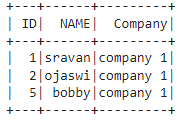## LeftAnti join

This  join returns only columns from the first dataframe for non-matched records of the second dataframe

Syntax: dataframe1.join(dataframe2,dataframe1.column_name ==  dataframe2.column_name,”leftanti”)

Example: In this example, we are going to perform leftanti join using leftanti keyword based on the ID column in both dataframes.

## Python3

 `# importing module``import` `pyspark`` ` `# importing sparksession from pyspark.sql module``from` `pyspark.sql ``import` `SparkSession`` ` `# creating sparksession and giving an app name``spark ``=` `SparkSession.builder.appName(``'sparkdf'``).getOrCreate()`` ` `# list  of employee data``data ``=` `[[``"1"``, ``"sravan"``, ``"company 1"``], ``        ``[``"2"``, ``"ojaswi"``, ``"company 1"``], ``        ``[``"3"``, ``"rohith"``, ``"company 2"``],``        ``[``"4"``, ``"sridevi"``, ``"company 1"``], ``        ``[``"5"``, ``"bobby"``, ``"company 1"``]]`` ` `# specify column names``columns ``=` `[``'ID'``, ``'NAME'``, ``'Company'``]`` ` `# creating a dataframe from the lists of data``dataframe ``=` `spark.createDataFrame(data, columns)`` ` `# list  of employee data``data1 ``=` `[[``"1"``, ``"45000"``, ``"IT"``],``         ``[``"2"``, ``"145000"``, ``"Manager"``],``         ``[``"6"``, ``"45000"``, ``"HR"``],``         ``[``"5"``, ``"34000"``, ``"Sales"``]]`` ` `# specify column names``columns ``=` `[``'ID'``, ``'salary'``, ``'department'``]`` ` `# creating a dataframe from the lists of data``dataframe1 ``=` `spark.createDataFrame(data1, columns)`` ` `# leftanti join on two dataframes``dataframe.join(dataframe1,``               ``dataframe.``ID` `=``=` `dataframe1.``ID``, ``               ``"leftanti"``).show()`

Output: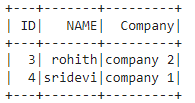## SQL Expression

We can perform all types of the above joins using an SQL expression, we have to mention the type of join in this expression. To do this, we have to create a temporary view.

Syntax: dataframe.createOrReplaceTempView(“name”)

where

• dataframe is the input dataframe
• name is the view name

Now we can perform join on these views using spark.sql().

Syntax: spark.sql(“select * from dataframe1, dataframe2 where dataframe1.column_name == dataframe2.column_name “)

where,

• dataframe1 is the first view dataframe
• dataframe2 is the second view dataframe
• column_name is the column to be joined

## Python3

 `# importing module``import` `pyspark`` ` `# importing sparksession from pyspark.sql module``from` `pyspark.sql ``import` `SparkSession`` ` `# creating sparksession and giving an app name``spark ``=` `SparkSession.builder.appName(``'sparkdf'``).getOrCreate()`` ` `# list  of employee data``data ``=` `[[``"1"``, ``"sravan"``, ``"company 1"``], ``        ``[``"2"``, ``"ojaswi"``, ``"company 1"``], ``        ``[``"3"``, ``"rohith"``, ``"company 2"``],``        ``[``"4"``, ``"sridevi"``, ``"company 1"``],``        ``[``"5"``, ``"bobby"``, ``"company 1"``]]`` ` `# specify column names``columns ``=` `[``'ID'``, ``'NAME'``, ``'Company'``]`` ` `# creating a dataframe from the lists of data``dataframe ``=` `spark.createDataFrame(data, columns)`` ` `# list  of employee data``data1 ``=` `[[``"1"``, ``"45000"``, ``"IT"``],``         ``[``"2"``, ``"145000"``, ``"Manager"``], ``         ``[``"6"``, ``"45000"``, ``"HR"``],``         ``[``"5"``, ``"34000"``, ``"Sales"``]]`` ` `# specify column names``columns ``=` `[``'ID'``, ``'salary'``, ``'department'``]`` ` `# creating a dataframe from the lists of data``dataframe1 ``=` `spark.createDataFrame(data1, columns)`` ` `# create a view for dataframe named student``dataframe.createOrReplaceTempView(``"student"``)`` ` `# create a view for dataframe1 named department``dataframe1.createOrReplaceTempView(``"department"``)`` ` `#use sql expression to select ID column``spark.sql(``    ``"select ``*` `from` `student, department\``    ``where student.``ID` `=``=` `department.``ID``").show()`

Output: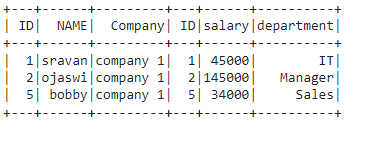We can also perform the above joins using this SQL expression:

Syntax: spark.sql(“select * from dataframe1 JOIN_TYPE dataframe2 ON dataframe1.column_name == dataframe2.column_name “)

where, JOIN_TYPE refers to above all types of joins

## Python3

 `# importing module``import` `pyspark`` ` `# importing sparksession from pyspark.sql module``from` `pyspark.sql ``import` `SparkSession`` ` `# creating sparksession and giving an app name``spark ``=` `SparkSession.builder.appName(``'sparkdf'``).getOrCreate()`` ` `# list  of employee data``data ``=` `[[``"1"``, ``"sravan"``, ``"company 1"``],``        ``[``"2"``, ``"ojaswi"``, ``"company 1"``],``        ``[``"3"``, ``"rohith"``, ``"company 2"``],``        ``[``"4"``, ``"sridevi"``, ``"company 1"``],``        ``[``"5"``, ``"bobby"``, ``"company 1"``]]`` ` `# specify column names``columns ``=` `[``'ID'``, ``'NAME'``, ``'Company'``]`` ` `# creating a dataframe from the lists of data``dataframe ``=` `spark.createDataFrame(data, columns)`` ` `# list  of employee data``data1 ``=` `[[``"1"``, ``"45000"``, ``"IT"``], ``         ``[``"2"``, ``"145000"``, ``"Manager"``], ``         ``[``"6"``, ``"45000"``, ``"HR"``],``         ``[``"5"``, ``"34000"``, ``"Sales"``]]`` ` `# specify column names``columns ``=` `[``'ID'``, ``'salary'``, ``'department'``]`` ` `# creating a dataframe from the lists of data``dataframe1 ``=` `spark.createDataFrame(data1, columns)`` ` `# create a view for dataframe named student``dataframe.createOrReplaceTempView(``"student"``)`` ` `# create a view for dataframe1 named department``dataframe1.createOrReplaceTempView(``"department"``)`` ` `# inner join on id column using sql expression``spark.sql(``    ``"select ``*` `from` `student INNER JOIN \``    ``department on student.``ID` `=``=` `department.``ID``").show()`

Output: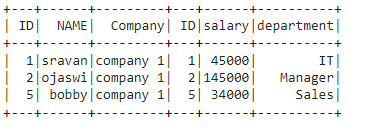My Personal Notes arrow_drop_up